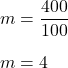## A 100 Newton force applied to a machine lifts a 400 N object. What is the actual mechanical advantage of this machine?

Question

A 100 Newton force applied to a machine lifts a 400 N object. What is the actual mechanical advantage of
this machine?

in progress 0
8 months 2021-07-19T00:54:06+00:00 1 Answers 0 views 0

## Answers ( )

m = 4

Explanation:

It is given that,

A 100 Newton force applied to a machine lifts a 400 N object.

We need to find the mechanical advantage of  this machine.

The mechanical advantage of a machine is given by the ratio of output force to the input force.

Here, output force is 400 N and input force is 100 N

So, mechanical advantage becomes :So, the mechanical advantage of this machine is 4.